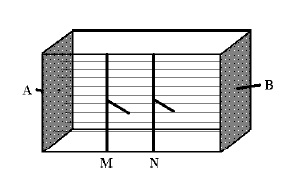Landviser, LLC Enlightening Research
 Search this site: this site has not been updated since 2017... visit us @ landviser.com  to learn about new products and services!

# Four-electrode probe

All the electrical resistivity methods applied in geophysics and soil science are based on the standard four-electrode principle suggested by Wenner in 1915 to minimize soil-electrode contact problems. The four-electrode principle is illustrated in the laboratory conductivity cell (Figure 1). The cell is a rectangular plastic box with the current electrodes A and B as brass plates on the smaller sides. The potential electrodes M and N are the brass rods in the middle of the long side of the cell. A constant current (I) is applied to the two outer electrodes (A and B) and the arising difference of potentials (ΔU) is measured between the two inner electrodes (M and N). The electrical resistivity (ER) is calculated from the Ohm's law aswhere K is a geometrical factor (m) depending on the distance among electrodes, AU is difference of potentials (mV), and I is magnitude of current (mA). The geometrical factor for a cell is obtained from the calibration solutions of a known resistivity (conductivity). The sample of soil paste or suspension is placed in a cell to measure electrical resistivity from the readings of voltage and current. The cell construction shown in Figure 1 ensures the induction of static uniform electrical field in the cell. The field is imposed on the homogeneous soil sample to measure an accurate electrical resistivity of a sample. The time variation and the difference in electrical resistivity are less than 0.5% when measured in the same soil sample by the cells with different distances between electrodes. The measurements in four-electrode laboratory cell were utilized to develop the relationships between various soil properties and electrical resistivity.Figure 1 Scheme of the four-electrode laboratory conductivity cell. Electrical field lines are shown with thin straight lines (uniform electrical field).